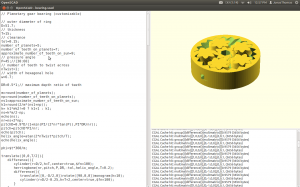# Trying to work on the Halloween project.

I’ve had this idea in my head for a halloween project.   I never leave myself enough time when halloween approaches…  Anyway.  I want to have a pumpking rotate and do a Linda Blair with some candy…

I had made a rotary table last year using gears and a lasy susan type bearing  and I was unhappy with the results.  Basically the bearing had way to much endplay..  I’m thinking I probably be better off with some type of belt instead of a gear mesh.

Recently, I was a trade show and I picked up on these gear bearings.

It’s actually pretty cool.   The gear bearing sample that I had was of much higher quality.

So one of the things I want to do is to hack this thing up and put a mounting flange and a belt cog on the ring gear and extend the sun gear to make a mounting shaft.

To get this into freecad, I’ve been told the way to do this fire up scad and load bearing.scad and then export it from scad as a csg and then import it into free cad… Some info here

At somepoint, if I ever have time, I’d love to get this converted into a freecad python script… Anyway… here’s what it looks like in scad.So… I went Design=>Export as Csg…   That went ok…  I then tried to import into freecad… Even with

“If FreeCAD crashes when importing CSG, it is strongly recommended that you enable ‘automatically check model after boolean operation’ in Menu -> Edit -> Preferences -> Part Design -> Model setting”

I was still getting crashes…. ;(  Oh well.

This is scad script which generates the gear…

// Planetary gear bearing (customizable)

// outer diameter of ring
D=51.7;
// thickness
T=15;
// clearance
tol=0.15;
number_of_planets=5;
number_of_teeth_on_planets=7;
approximate_number_of_teeth_on_sun=9;
// pressure angle
P=45;//[30:60]
// number of teeth to twist across
nTwist=1;
// width of hexagonal hole
w=6.7;

DR=0.5*1;// maximum depth ratio of teeth

m=round(number_of_planets);
np=round(number_of_teeth_on_planets);
ns1=approximate_number_of_teeth_on_sun;
k1=round(2/m*(ns1+np));
k= k1*m%2!=0 ? k1+1 : k1;
ns=k*m/2-np;
echo(ns);
nr=ns+2*np;
pitchD=0.9*D/(1+min(PI/(2*nr*tan(P)),PI*DR/nr));
pitch=pitchD*PI/nr;
echo(pitch);
helix_angle=atan(2*nTwist*pitch/T);
echo(helix_angle);

phi=\$t*360/m;

translate([0,0,T/2]){
difference(){
cylinder(r=D/2,h=T,center=true,\$fn=100);
herringbone(nr,pitch,P,DR,-tol,helix_angle,T+0.2);
difference(){
translate([0,-D/2,0])rotate([90,0,0])monogram(h=10);
cylinder(r=D/2-0.25,h=T+2,center=true,\$fn=100);
}
}
rotate([0,0,(np+1)*180/ns+phi*(ns+np)*2/ns])
difference(){
mirror([0,1,0])
herringbone(ns,pitch,P,DR,tol,helix_angle,T);
cylinder(r=w/sqrt(3),h=T+1,center=true,\$fn=6);
}
for(i=[1:m])rotate([0,0,i*360/m+phi])translate([pitchD/2*(ns+np)/nr,0,0])
rotate([0,0,i*ns/m*360/np-phi*(ns+np)/np-phi])
herringbone(np,pitch,P,DR,tol,helix_angle,T);
}

module rack(
number_of_teeth=15,
circular_pitch=10,
pressure_angle=28,
helix_angle=0,
clearance=0,
gear_thickness=5,
flat=false){

flat_extrude(h=gear_thickness,flat=flat)translate([0,-clearance*cos(pressure_angle)/2])
union(){
for(i=[1:number_of_teeth])
translate([circular_pitch*(i-number_of_teeth/2-0.5),0])
}
}

module monogram(h=1)
linear_extrude(height=h,center=true)
translate(-[3,2.5])union(){
difference(){
square([4,5]);
translate([1,1])square([2,3]);
}
square([6,1]);
translate([0,2])square([2,1]);
}

module herringbone(
number_of_teeth=15,
circular_pitch=10,
pressure_angle=28,
depth_ratio=1,
clearance=0,
helix_angle=0,
gear_thickness=5){
union(){
gear(number_of_teeth,
circular_pitch,
pressure_angle,
depth_ratio,
clearance,
helix_angle,
gear_thickness/2);
mirror([0,0,1])
gear(number_of_teeth,
circular_pitch,
pressure_angle,
depth_ratio,
clearance,
helix_angle,
gear_thickness/2);
}}

module gear (
number_of_teeth=15,
circular_pitch=10,
pressure_angle=28,
depth_ratio=1,
clearance=0,
helix_angle=0,
gear_thickness=5,
flat=false){

flat_extrude(h=gear_thickness,twist=twist,flat=flat)
gear2D (
number_of_teeth,
circular_pitch,
pressure_angle,
depth_ratio,
clearance);
}

module flat_extrude(h,twist,flat){
if(flat==false)
linear_extrude(height=h,twist=twist,slices=twist/6)child(0);
else
child(0);
}

module gear2D (
number_of_teeth,
circular_pitch,
pressure_angle,
depth_ratio,
clearance){
depth=circular_pitch/(2*tan(pressure_angle));
backlash_angle = clearance/(pitch_radius*cos(pressure_angle)) * 180 / PI;
half_thick_angle = 90/number_of_teeth – backlash_angle/2;
pitch_angle = atan2 (pitch_point, pitch_point);

intersection(){
rotate(90/number_of_teeth)
union(){
rotate(90/number_of_teeth)
for (i = [1:number_of_teeth])rotate(i*360/number_of_teeth){
halftooth (
pitch_angle,
half_thick_angle);
mirror([0,1])halftooth (
pitch_angle,
half_thick_angle);
}
}
}}

module halftooth (
pitch_angle,
half_thick_angle){
index=[0,1,2,3,4,5];
angle=index*(stop_angle-start_angle)/index[len(index)-1];
p=[[0,0],

difference(){
rotate(-pitch_angle-half_thick_angle)polygon(points=p);
}}

// Mathematical Functions
//===============

// Finds the angle of the involute about the base radius at the given distance (radius) from it’s center.
//source: http://www.mathhelpforum.com/math-help/geometry/136011-circle-involute-solving-y-any-given-x.html

// Calculate the involute position for a given base radius and involute angle.

[
1.pepe says: